This is chapter 9 of the "The Phenomenon of Science" by Valentin F. Turchin

Contents:

# CHAPTER NINE. MATHEMATICS BEFORE THE GREEKS

## NATURE'S MISTAKE

WE HAVE ALREADY mentioned the process of counting as an example of using a model of reality that is not contained in the brain but is created at the level of language. This is a very clear example. Counting is based on the ability to divide the surrounding world up into distinct objects. This ability emerged quite far back in the course of evolution; the higher vertebrates appear to have it in the same degree as humans do. It is plain that a living being capable of distinguishing separate objects would find it useful in the struggle for existence if it could also count them (for example, this would help one become oriented in an unfamiliar area). Description by means of numbers is a natural, integrated complement to differential description by recognition of distinct objects. Yet the cybernetic apparatus for recognizing numbers, for counting, can be extremely simple. This task is much easier than distinguishing among separate objects. Therefore one would expect that, within limits imposed by the organization of the organs of sight, recognition of numbers would have appeared in the course of evolution. The human eye can distinguish tens and hundreds of distinct objects at once. We might expect that human beings would be able to tell a group of 200 objects from a group of 201 just as easily as we tell two objects from three.

But nature did not wish or was unable to give us this capability. The numbers which are immediately recognizable are ridiculously few, usually four or five. Through training certain progress can be made, but this is done by mentally breaking up into groups or by memorizing pictures as whole units and then counting them in the mind. The limitation on direct discrimination remains. It is in no way related to the organization of the organs of sight and apparently results from some more deep-seated characteristics of brain structure. We do not yet know what they are. One fact forces us to ponder and suggests some hypotheses:

In addition to spatial discrimination of numbers there is temporal discrimination. You never confuse two knocks at a door with three or one. But eight or ten knocks is already, no doubt, ''many'' and we can only distinguish such sounds by their total length (this corresponds to the total area occupied by homogeneous objects in spatial discrimination). The limit which restricts both types of discrimination is the same. Is this a chance coincidence? It is possible that direct discrimination of numbers always has a temporal nature and that the capacity of the instantaneous memory limits the number of situations it can distinguish. In this case the limitation on spatial discrimination is explained by the hypothesis that the visual image is scanned into a time sequence (and there is a rapid switching of the eye's attention from object to object, which was discussed above) and is fed to the very same apparatus for analysis.

Be that as it may, nature has left an unfortunate gap in our mental device; therefore human beings begin work to create a "continuation of the brain" by correcting nature's mistake--humans learn to count, and thus mathematics begins.

## COUNTING AND MEASUREMENT

FACTS TESTIFY clearly that counting emerges before the names of the numbers. In other words, the initial linguistic objects for constructing a model are not words but distinct, uniform objects: fingers, stones, knots, and lines. That is natural. During the emergence of language, words refer only to those concepts which already exist, which is to say, those which are recognized. The words ''one," ''two,'' and possibly ''three'' appear independently of counting (taking "counting" to mean a procedure which is prolonged in time and recognized as such) because they rely on the corresponding neuronal concepts. There is as yet nowhere from which to take the words for large numbers. To convey the size of some group of objects, the human being uses standard objects, establishing a one-to-one correspondence among them, one after the other. This is counting. When counting becomes a widespread and customary matter, word designations begin to emerge for the most frequently encountered (in other words small) groups of standard objects. Traces of their origin have remained in certain numbers. For example, the Russian word for five, pyat,' is suspiciously similar to the old Slavic pyad,' which means hand (five fingers).

There are primitive peoples who have only ''one,'' ''two,'' and ''three''; everything else is "many.'' But this in no way excludes the ability to count by using standard objects, or to convey the idea of size by breaking down objects into groups of two or three, or by using as yet unreduced expressions, such as ''as many as the digits on two hands, one foot, plus one.'' The need for counting is simply not yet great enough to establish special words. The sequence ''one, two, three, many'' does not reflect an inability to count to four and beyond, as is sometimes thought, but rather a distinction the human mind makes between the first three numbers and all the rest. For we can only unconsciously--and without exertion--distinguish the numbers to three. To recognize a group of four we must concentrate especially. Thus it is true for us as well as for savages that everything which is more than three is ''many.''

To convey large numbers people began to count in "large units'': fives, tens, and twenties. In all the counting systems known to us large units are divisible by five, which indicates that the first counting tool was always the fingers. Still larger units arose from combinations of large units. Separate hieroglyphs depicting numbers up to ten million are found in Ancient Egyptian papyruses.

The beginning of measurement, just as with counting, goes back to ancient times. Measurement is already found among the primitive peoples. Measurement assumes an ability to count, and additionally it demands the introduction of a unit of measure and a measurement procedure that involves comparing what is being measured against a unit of measurement. The most ancient measures refer to the human body: pace, cubit [lit. ''elbow''; the unit was the length of the forearm], and foot.

With the emergence of civilization the need for counting and for the ability to perform mathematical operations increases greatly. In developed social production, the regulation of relations among people (exchange, division of property, imposition of taxes) demands a knowledge of arithmetic and the elements of geometry. And we find this knowledge in the most ancient civilizations known to us: Babylon and Egypt.

## NUMBER NOTATION

THE WRITING OF NUMBERS in ancient times demonstrates graphically the attitude toward the number as a direct model of reality. Let us take the Egyptian system for example. It was based on the decimal principle and contained hieroglyphs for the one (vertical line) and ''large ones.'' To depict a number it was necessary to repeat the hieroglyph as many times as it occurred in the number. Numbers were written in a similar way by other ancient peoples.Figure 9.1. Number notation by different ancient peoples. Adapted from G. I. Gleizer Istoriya matematiki v shkole (The History of Mathematics in School) Prosveshchenie Publishing House Moscow, 1964.

The Roman system is close to this very form of notation. It differs only in that when a smaller unit stands to the left of a larger it must be subtracted. This minor refinement (together with introduction of the intermediate units V, L, and D) eliminated the necessity of writing out a series of many identical symbols, giving the Roman system such vitality that it continues to exist to the present day.

An even more radical method of avoiding the cumbersome repetition of symbols is to designate key numbers (less than 10, then even tens, hundreds, and so on) by successive letters of the alphabet. This is precisely what the Greeks did in the eighth century B.C. Their alphabet was large enough for ones, tens, and hundreds; numbers larger than 1,000 were depicted by letters with a small slash mark to the left and beneath. Thus, [beta] signified two, [eta] signified 20, and /[beta] signified 2,000. Many peoples (such as the Armenians, the Jews, the Slavs) borrowed this system from the Greeks. With alphabetic nummeration the model form of the number completely disappears; it becomes merely a symbol. Simplification (for purposes of rapid writing) of characters which initially had model form leads to the same result.

"Arabic'' numerals are believed to be of Indian origin, although not all specialists agree with this hypothesis. Numbers are first encountered in Indian writings in the third century B.C. At this time two forms of writing were used, Kharoshti and Brahmi, and each one had its own numerals. The Kharoshti system is interesting because the number four was selected as the intermediate stage between 1 and 10. It is likely that the oblique cross (x) used as a 4 tempted the creators of the Kharoshti numbers by its simplicity of writing while still preserving the modeling quality in full (four rays). The Brahmi numerals are more economical. It is believed that the first nine Brahmi characters finally gave rise to our modern numerals.Figure 9.2. Kharoshti numerals.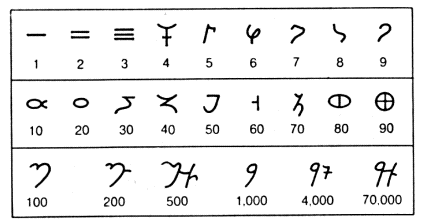Figure 9.3. Brahmi numerals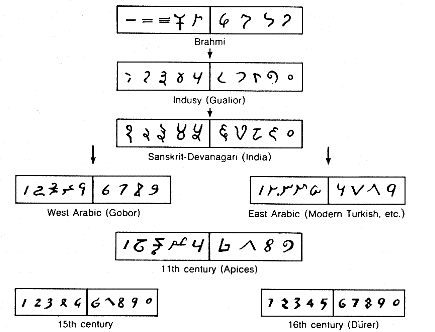Figure 9.4. The genealogy of modern numerals (according to Menninger, Zohlwort and Ziffer)

The loss of the model form in numbers was more than compensated for in the ancient world by the use of the abacus, a counting board with parallel grooves along which pebbles were moved. The different grooves corresponded to units of different worth. The abacus was probably invented by the Babylonians. It was used for all four arithmetic operations. Greek merchants used the abacus extensively and the same kind of counting board was common among the Romans. The Latin word for pebble, ''calculus,'' began also to mean "computation.'' And the Romans conceived the idea of putting the counting pebbles on rods, which is how the abacus still in use today originated. These very simple counting devices were enormously important and only gave way to computations on slateboards or paper after the positional system of notation had completely formed.

## THE PLACE-VALUE SYSTEM

THE BABYLONIANS laid the foundations of the place-value system the number system they borrowed from the Sumerians, we see two basic ''large ones'': ten and sixty, from the most ancient clay tablets which have come down to us, dating to the beginning of the third millennium B.C. We can only guess where the number sixty was taken from. The well-known historian of mathematics O. Neigebauer believes that it originated in the relation between the basic monetary units in circulation in Mesopotamia: one mana (in Greek mina was sixty shekels). Such an explanation does not satisfy our curiosity because the question immediately arises: why are there sixty shekels in a mana? Isn't it precisely because a system based on sixty was used? After all, we don't count by tens and hundreds because there are 100 kopecks in a ruble! F. Thureau-Dangin, an Assyriologist, gives linguistic arguments to show that the number system was the primary phenomenon and the system of measures came second. Selection of the number sixty was apparently a historical accident, but one can hardly doubt that this accident was promoted by an important characteristic of the number sixty, namely that it has an extraordinarily large number of divisors 2, 3, 4, 5, 6, 10, 12, 15, 20, and 30). This is a very useful feature both for a monetary unit (since its existent money has been evenly subdivided) and for establishing a system of counting (if we assume that some wise man introduced it, guided considerations of convenience in calculation).

The mathematical culture of the Babylonians is known to us from texts dating from the Ancient Babylonian (1800-1600 B.C.) and the Seleucidae epoch (305-64 B.C.). A comparison of these texts shows that no radical changes took place in the mathematics of the Babylonians during this time.

The Babylonians depicted 1 by a narrow vertical wedge --, while 10 was a wide horizontal wedge.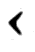The number 35 looked like this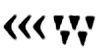All numbers up to 59 were represented analogously. But 60 was depicted once again by a narrow vertical wedge, just the same as 1. In the most ancient tablets it can be seen that the wedge representing 60 is larger than the wedge for 1. thus the number 60 was not only understood as a "larger one" but was so represented. "Large tens" appeared correspondingly for large units multiplied by 10. Later, the difference between large and small wedges was lost and they began to be distinguished by their postion. In this way the positional system arose. A Babylonian would write the number 747 = 12 X 60 + 27 in the form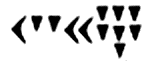The third 60-base position corresponds to the number 602 = 3,600 and so on. But the most remarkable thing is that the Babylonians also represented fractions in this way. In a number following the number of ones, each unit signified 1/60, in the next number each unit was 1/3,600, and so on. In modern decimal notation we separate the whole part from the fraction part by a period or comma. The Babylonians did not. The number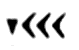could signify 1.5 or 90 with equal success. This same uncertainty occured in writing whole numbers: the numbers n, n X 60, n X 602, and so on were indistinguishable. Multipliers and divisors divisible by 60 had to be added according to the sense. Because 60 is a fairly large number, this did not cause any particular problems.

When we compare the Babylonian positional system with our modern one we see that the uncertainty in the multiplier 60 is a result of the absence of a character for zero, which we would add the necessary number of times at the end of a whole number or the beginning of a fraction. Another result of the absence of the zero is an even more serious uncertainty in interpreting a numerical notation that in our system requires a zero in an intermediate position. In the Babylonian notation, how can the number 3,601 = 1 . 602 + 0 . 60 + 1 be distinguished from the number 61 = 1. 60 + 1 ? Both of these numbers are represented by two units (ones). Sometimes this kind of uncertainty was eliminated by separating the numbers, leaving an empty place for the missing position. But this method was not used systematically and in many cases a large gap between numbers did not mean any thing. In the astronomical tables of the Seleucidae epoch one finds the missing position designated by means of a character resembling our period. We do not find anything of the sort in the Ancient Babylonian epoch. But how were the ancient Babylonians able to avoid confusion?

The solution to the riddle is believed to consist in the following. The early Babylonian mathematical texts which have come down to us are collections of problems and their solutions, unquestionably created as learning aids. Their purpose was to teach practical methods of solving problems. But not one of the texts describes how to perform arithmetic operations, in particular the operations, complex for that time, of multiplication and division. Therefore, we assume that the students knew how to do them. It is improbable that they performed the computations in their head: they probably used some abacus-like calculating device. On the abacus, numbers appear in their natural, spontaneously positioned form and no special character for the zero is needed; the groove corresponding to an empty position simply remains without pebbles. Representation of a number on the abacus was the basic form of assigning a number, and there was no uncertainty in this representation. The numbers given in cuneiform mathematical texts serve as answers to stage-by-stage calculations, so that they could be used to check correctness during the solution. The student made the calculations on the abacus and checked them against the clay tablet. Clearly the absence of a character for empty positions did not hinder such checking at all. When voluminous astronomical tables became widespread and were no longer used for checks but rather as the sole source of data, a separation sign began to be used to represent the empty positions. But the Babylonians never put their own ''zero'' at the end of a word; it is obvious that they perceived it only as a separator, and not as a number.

Having familiarized themselves with both the Egyptian and the Babylonian systems of writing fractions and performing operations on them, the Greeks selected the Babylonian system for astronomical calculations because it was incomparably better, but they preserved their own alphabetic system for writing whole numbers. Thus the Greek system used in astronomy was a mixed one: the whole part of the number was represented in the decimal nonpositional system while the fractional part was in the 60-base positional system--not a very logical solution by the creators of logic! Following their happy example we continue today to count hours and degrees (angular) in tens and hundreds, but we divide them into minutes and seconds.

The Greeks did introduce the modern character for zero into the positional system, deriving it--in the opinion of a majority of specialists--from the first letter of the wo doudeg, which means ''nothing." In writing whole numbers (except for the number 0) this character was not used, naturally, because the alphabetic system which the Greeks used was not a positional one.

The modern number system was invented by the Indians at the beginning of the sixth century A.D. They applied the Babylonian positional principle and the Greek character for zero to designate an empty place to a base of 10, not 60. The system proved to be consistent, economical, not in contradiction with tradition, and extremely convenient for computations.

The Indians passed their system on to the Arabs. The positional number system appeared in Europe in the twelfth century with translations of al-Kwarizmi's famous Arab arithmetic. It came into bitter conflict with the traditional Roman system and in the end won out. As late as the sixteenth century, however, an arithmetic textbook was published in Germany and went through many editions using exclusively ''German,'' which is to say Roman, numerals. It would be better to say ''numbers,'' because at that time the word ''numerals" was used only for the characters of the Indian system. In the preface of this textbook the author writes: ''I have presented this arithmetic in conventional German numbers for the benefit and use of the uneducated reader (who will find it difficult to learn numerals at the same time).'' Decimal fractions began to be used in Europe with Simon Stevin (1548-1620).

## APPLIED ARITHMETIC

THE MAIN LINE to modern science lies through the culture of Ancient Greece, which inherited the achievements of the ancient Egyptians and Babylonians. The other influences and relations (in particular the transmission function carried out by the Arabs) were of greater or lesser importance but, evidently, not crucial. The sources of the Egyptian and Sumerian-Babylonian civilizations are lost in the dark of primitive cultures. In our review of the history of science, therefore, we shall limit ourselves to the Egyptians, Babylonians, and Greeks .

We have already discussed the number notation of the Egyptians and Babylonians. All we need now is to add a few words about how the Egyptians wrote fractions. From a modern point of view their system was very original, and very inconvenient. The Egyptians had a special form of notation used only for so-called 'basic'' fractions. that is, those obtained by dividing one by a whole number; in addition they used two simple fractions which had had special hieroglyphs from ancient times: 2/3 and 3/4. In the very latest papyruses, however, the special designation for 3/4 disappeared. To write a basic fraction the symbol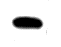which meant "part," had to be placed above the conventional number (the denominator). ThusThe Egyptions expanded the other fractions into the sum of several basic fractions. For example, 3/8 was written as 1/4 + 1/8, and 2/7 was written as 1/4 + 1/ 28. For the result of dividing 2 by 29 an Egytian table gave the following expansion:

2/29 = 1/29 + 1/58 = 1/174 + 1/232.

We are not going to dwell on the computational techniques of the Egyptians and Babylonians. It is enough to say that they both were able to perform the four arithmetic operations on all numbers (whole, fractional, or mixed) which they met in practice. They used auxiliary mathematical tables for operations with fractions; these were tables of inverse numbers among the Babylonians and tables of the doubling of basic fractions among the Egyptians. The Egyptians wrote intermediate results on papyrus, whereas the Babylonians apparently performed their operations on an abacus and thus the details of their technique remain unknown.

What did the ancient mathematicians calculate? One fragment of an Egyptian papyrus from the times of the New Empire (1500-500 B.C.) describes the activity of the pharaoh's scribes very colorfully and with a large dose of humor; for this reason it is invariably cited in we shall not be an exception. Here is the excerpt, somewhat shortened:

I will cause you to know how matters stand with you, when you say "I am the scribe who issues commands to the army. ". . . I will cause you to be abashed when I disclose to you a command of your lord, you, who are his Royal Scribe.... the clever scribe who is at the head of the troops. A building ramp is to be constructed, 730 cubits long, 55 cubits wide, containing 120 compartments, and filled with reeds and beams; 60 cubits high at its summit, 30 cubits in the middle, with a batter of twice 15 cubits and its pavement 5 cubits. The quantity of bricks needed for it is asked of the generals, and the scribes are all asked together, without one of them knowing anything. They all put their trust in you and say "You are the clever scribe. my friend! Decide for us quickly!" Behold your name is famous.... Answer us how many bricks are needed for it?

Despite its popularity, this text is not too intelligible. But it nevertheless does give an idea of the problems Egyptian scribes had to solve.

Specifically, we see that they were supposed to be able to calculate areas and volumes (how accurately is another question). And in fact, the Egyptians possessed a certain knowledge of geometry. According to the very sound opinion of the Ancient Greeks, this knowledge arose in Egypt itself. One of the philosophers of Aristotle's school begins his treatise with the words:

Because we must survey the beginning of the sciences and arts here we will state that, according to the testimony of many, geometry was discovered by the Egyptians and originated during the measurement of land. This measurement was necessary because the flooding of the Nile River constantly washed away the boundaries. There is nothing surprising in the fact that this science, like others, arose from human need. Every emerging knowledge passes from incomplete to complete. Originating through sensory perception it increasingly becomes an object of our consideration and is finally mastered by our reason. 

The division of knowledge into incomplete and complete and a certain apologetic tone concerning the "low'' origin of the science are, of course, from the Greek philosopher. Neither the Babylonians nor the Egyptians had such ideas. For them knowledge was something completely homogeneous. They were able to make geometric constructions and knew the formulas for the area of a triangle and circle just as they knew how to shoot bows and knew the properties of medicinal plants and the dates of the Nile's floods. They did not know geometry as the art of deriving "true" formulas; among them it existed, as B. Van der Waerden expressed it, only as a division of applied arithmetic. It is obvious that they employed certain guiding considerations in obtaining the formulas, but these considerations were of little interest to them. They did not affect their attitude toward the formula.

## THE ANCIENTS' KNOWLEDGE OF GEOMETRY

WHAT GEOMETRY did the Egyptians know?--the correct formulas for the area of a triangle, a rectangle, and a trapezium. The area of an irregular quadrangle, to judge by the one remaining document, was calculated as follows: half the sum of two opposite sides was multiplied by half the sum of the other two opposite sides. This formula is grossly wrong (except where the quadrangle is rectangular, in which case the formula is unnecessary). There is no reasonable sense in which it can even be called approximate. It appears that this is the first historically recorded example of a proposition which is derived from "general considerations," not from a comparison with the data of experience. The Egyptians calculated the area of a circle by squaring 8/9, of its diameter, a difference of about 1 percent from the value of pi.

They calculated the volumes of parallelepipeds and cylinders by multiplying the area of the base by the height. The most sophisticated achievement of Egyptian geometry known to us is correct computation of the volume of a truncated pyramid with a square base (the Moscow papyrus). It follows the formula

V=( a2 +ab +b2) h/3

where h is the height, a and b are the sides of the upper and lower bases.

We have only fragmentary information on the Ancient Babylonians' knowledge of mathematics, but we can still from a general idea of it. It is completely certain that the Babylonians were aware of what came to be called the ''Pythagorean theorem''--the sum of the squares of the sides of a right triangle is equal to the square of the hypotenuse. Like the Egyptians they computed the areas of triangles and trapeziums correctly. They computed the circumference and area of a circle using a value of [pi]=3, which is much worse than the Egyptian approximation. The Babylonians calculated the volume of a truncated pyramid or cone by multiplying half of the sum of the areas of the bases by the height (an incorrect formula).

## A BIRD'S EYE VIEW OF ARITHMETIC

THE SITUATIONS and representations in the human nervous system model the succession of states of the environment. Linguistic objects model the succession of situations and representations. As a result a theory is a ''two-story'' linguistic model of reality.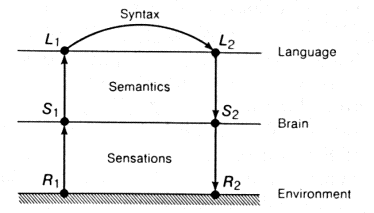Figure 9.5. Diagram of the use of a linguistic model of reality

This diagram shows the use of a theory. The situation S1 is encoded by linguistic object L1. This object may, of course, consist of a set of other objects and may have a very highly complex structure. Object L1 is the name for S1. Sometime later situation S1 changes into situation S2. A certain linguistic activity is performed and we convert L1 into an other object L2; if our model is correct L2 is the name of S2. Then, without knowing the real situation S2, we can get an idea of it by decoding linguistic object L2. The linguistic model is plainly determined by both the semantics of objects L1 (the "material part'' according to Russian military terminology) and the type of linguistic activity which converts L1 into L2.

Notice that we have not said anything about "isolating the essential aspects of the phenomenon," ''the cause-effect relation," or other such things which are usually set in places of honor when describing the essence of scientific modeling. And in our presentation, situation S1 does not "generate" situation S2 but only ''changes into'' it. Of course, this is no accident. The diagram we have drawn logically precedes the above-mentioned philosophical concepts. If we have a linguistic model (and only to the extent that we do have one) we can talk about the essential aspect of a phenomenon, idealization, the cause-effect relation, and the like. Although they appear to be conditions for the creation of a linguistic model, all of these concepts are in fact nothing but description in general terms (although very important and necessary ones) of already existing models. Although these concepts appear to ''explain'' why a linguistic model can exist in general, in reality they are elements of a linguistic model of the next level of the control hierarchy and, of course, appear later in history than the primary linguistic models (for example arithmetic ones). Before using these concepts, therefore, we must ascertain that linguistic models exist in general. And on this level of description we need not add anything to the diagram shown in figure 9.5.

But theories are created and developed by the trial and error method. If there is a starting point, then beginning from it a person tries to build linguistic constructions and test the results. The phases of building and testing are constantly alternating: construction gives rise to testing and testing gives rise to new constructions.

The starting point of arithmetic is the concept of the whole number. The aspect of reality this concept reflects is the following: the relation of the whole to its parts, the procedure for breaking the whole down into parts. The same thought can be expressed the other way around: a number is a procedure for joining parts into a whole, that is, into a certain set. Two numbers are considered identical if their parts (set elements) can be placed in a one-to-one correspondence; establishing this correspondence is counting. It is obvious, however, that numbers are not enough for a theory; we must also have operations with them. These are the elements of the model's functioning, the conversions L1 -> L2. Let us take two numbers n and m and represent them schematically as two modes of breaking a whole down into parts (figure 9.6 a).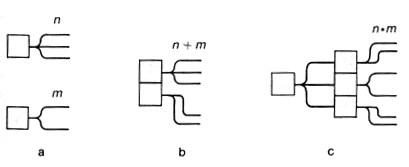Figure 9.6. Operations on whole numbers

How can we from these two numbers obtain a third--that is, a third mode of breaking down the whole into parts? Two modes come to mind immediately. They can be called parallel and sequential joining of breakdowns. In the parallel mode both wholes form parts of a new whole (figure 9.6 b). This breakdown (number) we call the sum of the two numbers. With the sequential mode we take one of the breakdowns and break down each of its parts in accordance with the other breakdown (figure 9.6 c). The new number is called the product. It does not depend on the order of the generating numbers. This can be seen very well if we interpret the actions with the numbers not as joining breakdowns but as forming a new set. The sum is obviously the result of merging the two sets into one (their union). The prototype of the product is the set of combinations of any element of the first set with any element of the second (in mathematics such a set is called the direct product of sets). The connection between this definition and the preceding one can be traced as follows. Suppose the first breakdown divides whole number A into parts a1, a2, . . ., and the second divides B into parts b1, b2, . . . , bm . After performing the first breakdown we mark the parts obtained with the letters ai. Breaking down each part into parts bjwe keep the first letter and add a second. This means that in each part of the result there will be an aj abj, and all these combinations will be different.

The approaches from the whole to the part and from the part to the whole complement one another. It is also easy to see from figure 9.6 c that multiplication can be reduced to repeated addition. Of course the ancients who were creating arithmetic were far from this reasoning. But then again, the frog did not know that its nervous system had to be organized on the hierarchical principle either! What is important is that we know this.

Having linguistic objects that depict numbers and being able to perform addition and multiplication with them we receive a theory that gives us working models of reality. Let us figure a very simple example, which clarifies the diagram in figure 9.6.

Suppose a certain farmer has planted wheat in a field 60 paces long and 25 paces wide. We shall assume that the farmer expects a yield of one bushel of wheat per square pace. Before beginning the harvest he wants to know how many bushels of wheat he will get. In this case S1 is the situation before the wheat harvest, specifically including the result of measuring the width and length of the field in paces and the expected yield; S2 is the situation after the harvest, specifically including the result of measuring the amount of wheat in bushels; L1 is the linguistic object 60 x 25 (the multiplication sign is a reflection of situation S1 just as the numbers 60 and 25 are; it reflects the structure of the set of square paces on the plane as a direct product of the sets of linear paces for length and width); S2 is the linguistic object 1.500.

Note that by theory we mean simply a linguistic model of reality which gives something new in comparison with neuronal models. This definition does not take into account that theories may form a control hierarchy; this fact is difficult to reflect without introducing mathematical apparatus. More general models can generate more particular ones. We shall consider the terms theory and linguistic model to be synonymous, but nonetheless when we are speaking of one model generating another, we shall call the more general one a theory and the more particular one a model.

## REVERSE MOVEMENT IN A MODEL

A THEORY THAT has just been created must first be tested comprehensively. It must be compared with experience and searched for flaws. If the theory is valid, an attempt must then be made to give the model ''reverse movement,'' to determine specific characteristics of L1 on the basis of a given L2. This procedure is by no means without practical importance. A person uses a model for planning purposeful activity and wants to know what he must do to obtain the required result--what L1 should be in order to obtain a given L2. In our example with the farmer, the question can be put as follows: given the width of a certain field, what should the length be to obtain a given amount of wheat?

But studying the reverse movement of a model is not always dictated by practical needs of the moment. Often it is done for pure curiosity--to "see what happens.'' Nonetheless, the result of such activity will be a better understanding of the organization and characteristics of the model and the creation of new constructions and models which, in the last analysis, will lead to greatly enlarged practical usefulness. This is the supreme wisdom of nature, which gave human beings ''pure'' curiosity.

In arithmetic the reverse movement of a model leads to the concept of the equation. The simplest equations generate the operations of subtraction and division. Using modern algebraic language. we define the difference b--a as the solution of the equation a + x = b-- in which x is the number that makes this equality true. The quotient from dividing b by a is determined analogously. The operation of division generates a new construction: the fraction. Repeated multiplication of a number by itself generates the construction of exponential degree, and the reverse movement generates the operation of extracting the root. This completes the list of arithmetic constructions in use among the Ancient Egyptians and Babylonians.

## SOLVING EQUATIONS

WITH THE DEVELOPMENT of techniques of computation, and with the development of civilization in general, increasingly complex equations began to appear and to be solved. The ancients, of course, did not know modern algebraic language. They expressed equations in ordinary conversational language as is done in our grammar school arithmetic textbooks. Nevertheless, their substance was not changed. The ancients (and today's school children) were solving equations.

The Egyptians called the quantity subject to determination the akha which is translated as ''certain quantity'' or ''bulk.'' Here is an example of the wording of a problem from an Egyptian papyrus: "A quantity and its fourth part together give 15.'' In modern mathematical terminology this is the problem of ''parts,'' and in algebraic language it corresponds to the equation x + 1/4 x = 15.

Let us give an example of a more complex problem from Egyptian times. ''A square and another square whose side is 1/2 + 1/4 of a side of the first square together have an area of 100. Calculate this for me.'' The solution in modern notation is as follows:

x2 + (3/4 x)2 = 100

(1 + 9/16)x2 = 100

5/4 x= 10

x = 8, 3/4 x = 6

Here is the description of the solution in a papyrus:

"Take a square with side I and take 1/2 + 1/4 of 1, that is, 1/2 + 1/4, as the side of the second area. Multiply 1/2 + 1/4 by itself; this gives 1/2 + 1/l6. Because the side of the first area is taken as 1 and the second as 1/2 + 1/4, add both areas together; this gives 1 + 1/2 + 1/16. Take the root from this: this will be 1 + 1/4. Take the root of the given 100: this will be 10. How many times does 1 +1/4 go into 10? It goes eight times.''

The rest of the text has not been preserved, but the conclusion is obvious: 8 . 1 = 8 is the side of the first square and 8 . (1/2 + 1/ 4) = 6 is the second.

The Egyptians were able to solve only linear and very simple quadratic equations with one unknown. The Babylonians went much further. Here is an example of a problem from the Babylonian texts:

''I added the areas of my two squares: 25 . 25/60. The side of the second square is 2/3 of the side of the first and five more." This is followed by a completely correct solution of the problem. This problem is equivalent to a system of equations with two unknowns:

x2 + y2 = 25 . 25/60

y = 2/3 x + 5

The Babylonians were able to solve a full quadratic equation:

x2 +- ax = b,

cubic equations:

x3 = a

x2 (x + 1 ) = a

and other systems of equations similar to those given above as well as ones of the type

x +- y = a, Xy = b

In addition to this they used formulas

(a + b)2 = a2 + 2ab + b2

(a + b)(a-b) = (a2 - b2)

were able to sum arithmetic progressions, knew the sums of certain number series, and knew the numbers which later came to be called Pythagorian (such whole numbers x, y, and z that x2 + y2 = z2 ).

## THE FORMULA

THE PLACE of Ancient Egypt and Babylon in the history of mathematics can be defined as follows: the formula first appeared in these cultures. By formula we mean not only the alphanumeric expression of modern algebraic language but in (general any linguistic object which is an exact (formal) prescription for how to make the conversion L1 -> L2, or any auxiliary conversions within the framework of language. Formulas are a most important part of any elaborated theory although, of course, they do not exhaust it because a theory also includes the meanings of linguistic objects Li. The assertion that there is a relation between the magnitudes of the sides in a right triangle. which is contained in the Pythagorian theorem, is a formula even though it is expressed by words rather than letters. A typical problem with a description of the process of solution ("Do it this way!") and with a note that the numbers may be arbitrary (this may not be expressed but rather assumed) is also a formula. It is precisely such formulas which have come down to us in the Egyptian papyruses and the Babylonian clay tablets.

 See B. L. van der Waerden's book Ontwakende Wetenschap, in English: Science Awakening, New York: Oxford University Press, 1969).

 van der Waerden, Science Awakening, p. 17.

 This fragment has come down to us through Procul (fitth century B.C.), a commentator on Euclid.